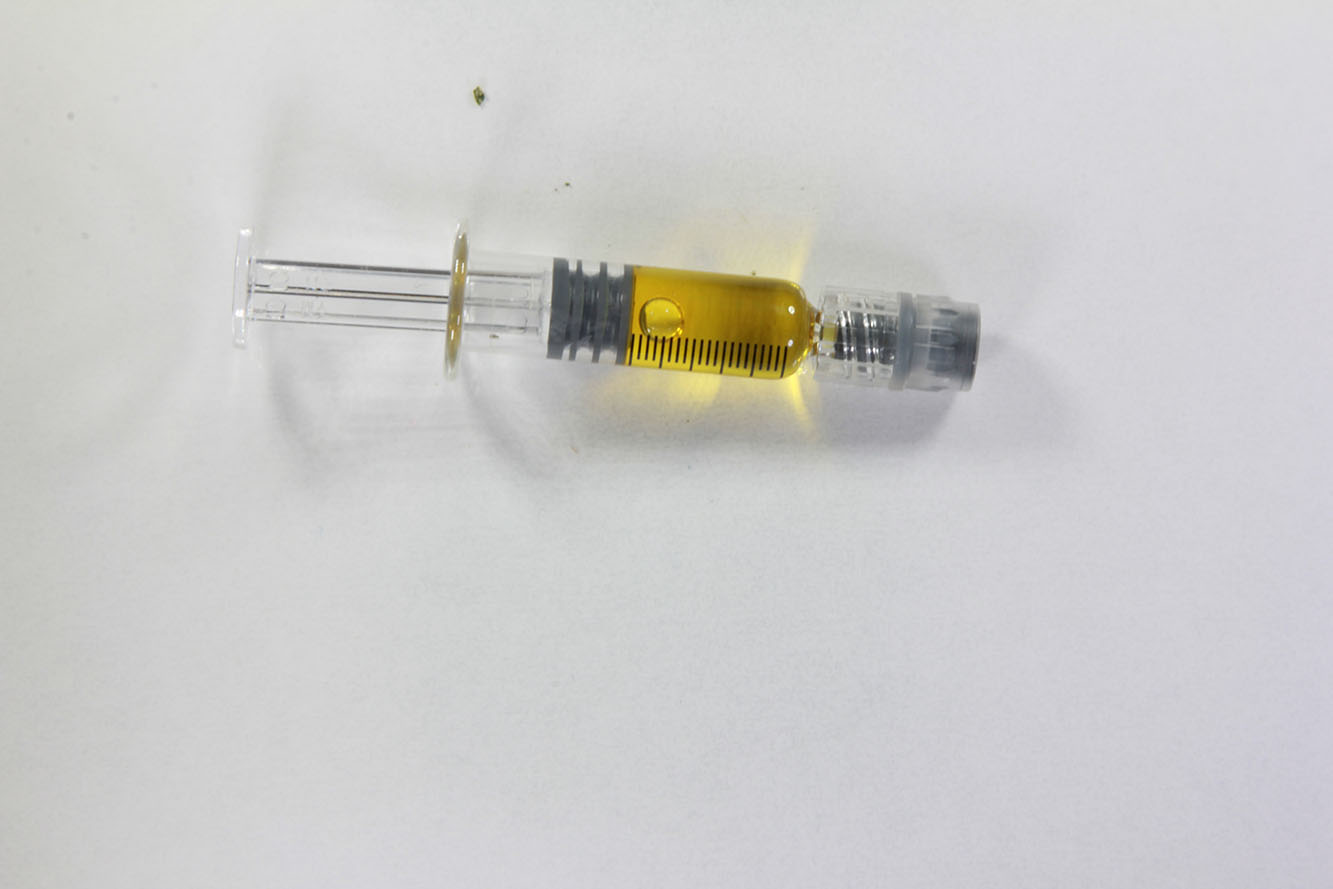### 88.48%

Total Cannabinoids

### -

Total Terpenes
Tested 12/19/2022 for Potency by MCR Labs Massachusetts

### POTENCY PROFILES

#### Cannabinoid Profile

88.48%
Total Cannabinoids
82.6%
Max THC
0.29%
Max CBD

Cannabinoids are quantified using high-performance liquid chromatography.

Total Cannabinoids is the sum of all detected cannabinoids.

Max THC THCa is converted to THC by heat. Max THC is the maximum amount of THC that can be derived from this product. Use the following formula to find the Max THC: Max THC = THC + (THCa * 0.877)

Max CBD CBDa is converted to CBD by heat. Max CBD is the maximum amount of CBD that can be derived from this product. Use the following formula to find the Max CBD: Max CBD = CBD + (CBDa * 0.877)

Other* This represents materials other than cannabinoids in the product.

To determine how much Distillate to consume to achieve your desired dose, use the:

mg
﻿﻿